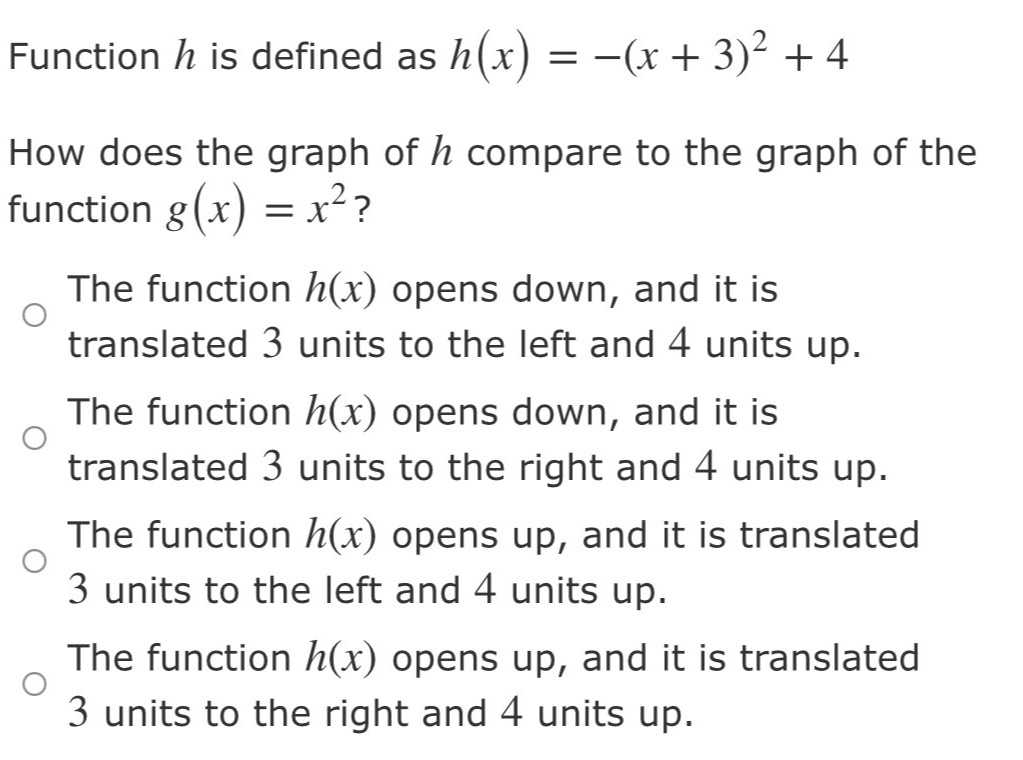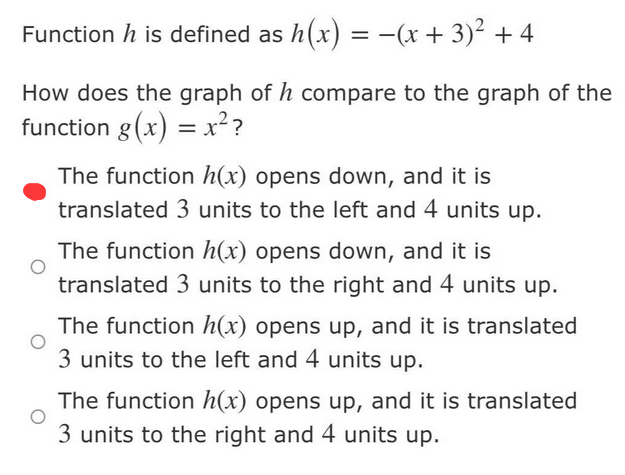### Still have math questions?

Algebra
QuestionFunction $$h$$ is defined as $$h ( x ) = - ( x + 3 ) ^ { 2 } + 4$$

How does the graph of $$h$$ compare to the graph of the function $$g ( x ) = x ^ { 2 }$$ ? The function $$h ( x )$$ opens down, and it is translated $$3$$ units to the left and $$4$$ units up. The function $$h ( x )$$ opens down, and it is translated $$3$$ units to the right and $$4$$ units up. The function $$h ( x )$$ opens up, and it is translated

$$3$$ units to the left and $$4$$ units up. The function $$h ( x )$$ opens up, and it is translated

$$3$$ units to the right and $$4$$ units up.